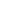# Exploring General Chemistry in the Laboratory

By Colleen F. Craig, Kim N. Gunnerson  •
2017
•  592
Pages
Loose-leaf
Format •
Print ISBN  9781617316227
• eBook ISBN  9781617316234Suggested Student Retail Price

\$96.80

PurchaseRequest review copy
###### eBook Options

This laboratory manual is intended for a two-semester general chemistry course. The procedures are written with the goal of simplifying a complicated and often challenging subject for students by applying concepts to everyday life.

This lab manual covers topics such as composition of compounds, reactivity, stoichiometry, limiting reactants, gas laws, calorimetry, periodic trends, molecular structure, spectroscopy, kinetics, equilibria, thermodynamics, electrochemistry, intermolecular forces, solutions, and coordination complexes.

By the end of this course, you should have a solid understanding of the basic concepts of chemistry, which will give you confidence as you embark on your career in science.

Examples of interior pages:Features
Instructor Resources
Features
• INITIATE your study of the basic concepts in the general chemistry laboratory by reading through concise introductory material and answering pre-lab questions that familiarize you with the steps in each exercise.
• INVESTIGATE the mysteries of matter by following the clearly written procedures and recording data and observations on the data sheets provided. Note boxes, OSHA pictograms, and precise explanations of techniques help you develop laboratory skills while safely working through procedures.
• INTEGRATE your knowledge of each laboratory topic by making sense of the data you have collected. Critical thinking post-lab questions assist you in understanding what you have performed in lab.
Introduction to the Chemistry Laboratory
Chapter 1: Measurement
Chapter 1 Intro Material
Lab 1.1 Temperature and Density: The Galileo Thermometer
Lab 1.2 Conservation of Mass
Chapter 2: Composition and Reactivity of Compounds
Chapter 2 Intro Material
Lab 2.1 Composition of Chemical Compounds
Lab 2.2 Reactivity of Hydrogen and Oxygen Gases
Chapter 3: Stoichiometry
Chapter 3 Intro Material
Lab 3.1 Acid-Base Titration
Lab 3.2 Limiting Reactants
Chapter 4: Behavior of Gases
Chapter 4 Intro Material
Lab 4.1 Avogadro's and Boyle's Laws
Lab 4.2 Density of a Gas
Chapter 5: Thermochemistry
Chapter 5 Intro Material
Lab 5.1 Coffee Cup Calorimetry
Lab 5.2 Hess' Law of Heat Summation
Chapter 6: Atomic Emission Spectra and Periodic Trends
Chapter 6 Intro Material
Lab 6.1 Atomic Emission Spectra
Lab 6.2 Periodic Trends
Chapter 7: Molecular Structure and its Consequences
Chapter 7 Intro Material
Lab 7.1 Molecules in 3-D
Lab 7.2 Visible Absorption Spectroscopy
Chapter 8: Chemical Kinetics
Chapter 8 Intro Material
Lab 8.1 Method of Initial Rates
Lab 8.2 Integrated Rate Laws
Chapter 9: Chemical Equilibrium
Chapter 9 Intro Material
Lab 9.1 Equilibrium of Bromothymol Blue
Lab 9.2 Solubility Product Constant of Potassium Hydrogen Phthalate
Chapter 10: Aqueous Equilibrium
Chapter 10 Intro Material
Lab 10.1 Titration Curves of Acids and Bases
Lab 10.2 Buffer Solutions
Chapter 11: Thermodynamics
Chapter 11 Intro Material
Lab 11.1 Determination of ∆H° and ∆S° Using Spectroscopy
Lab 11.2 Determination of ∆H° and ∆S° Using Titration
Chapter 12: Electrochemistry
Chapter 12 Intro Material
Lab 12.1 Galvanic Cells
Lab 12.2 Electroplating and Electrolysis
Chapter 13: Consequences of Intermolecular Forces
Chapter 13 Intro Material
Lab 13.1 Viscosity
Lab 13.2 Surface Tension and Capillary Action
Chapter 14: Properties of Solutions
Chapter 14 Intro Material
Lab 14.1 Freezing Point Depression
Lab 14.2 Vapor Pressure of Solutions
Chapter 15: Coordination Complexes
Chapter 15 Intro Material
Lab 15.1 Titration Curves of Acids and Bases
Lab 15.2 Spectrochemical Series and Crystal Field Theory
Chapter 16: Organic and Biological Molecules
Chapter 16 Intro Material
Lab 16.1 Thin Layer Chromatography of Spinach
Lab 16.2 Synthesis of Aspirin
Appendices
Appendix A: Mathematical Techniques
Appendix B: Titration
Appendix C: Spectroscopy
Appendix D: Beer's Law and Calibration Curves
Appendix E: Organic Molecular Structure
Appendix F: Alternate Procedure for Lab Activity 6.1
Appendix G: Reference Equations
Index